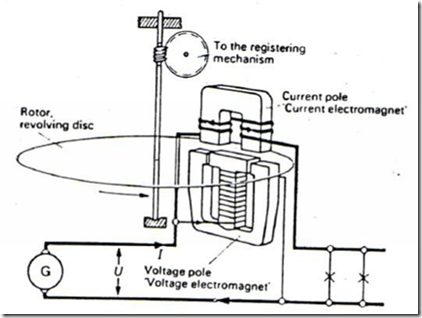Home > English > Configuration Measurement Tool Kwh Meter

# Configuration Measurement Tool Kwh Meter

KWH Meter is used to record the amount of electrical energy used by a power load be it resistive, inductive, capacitive. Everything will be recorded by the KWH Meter tool. Based on how it works (design) KWH Meter is divided into two parts, namely:
1. KWH meter with motor prinsif,
2. KWH meter with static meter.

Static meters are used for direct current meters. While KWH meter with motor principle used for meter induction of alternating current (alternating current). The motorized kWH meter has a rotor without a coil made of aluminum disks, which is positioned between two electric magnetic poles. Namely poles of current coils and poles of voltage coilsFigure 55 KWH Meter with Prinsif Motor

The number of rotations of the rotor in per KWH is called the meter constants or (C), which is usually listed on the KWH meter nameplate itself. Suppose we take the example: 600U / KWH (600 turns per KWH); Or 1200U / KWH (meaning 1200 revolutions per KWH) And so on. In this case KWH meter is used as a tool to measure the active power (wattage power) of an electrical load. therefore KWH meter can also be used to determine the amount of power (watts) of the load as for the formula of the formula used in the KWH meter theory is as follows:
P =(KW)
Where :
P = Active Power (Watt)
n = Number of rounds per minute from disc
C = Meter constant"Tasikmalaya, Indonesia"